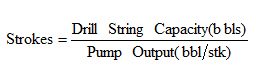## Well Control Formulas Part 3

This is the 3rd part of well control formulas.Annulus Capacity Factor (ACF),bbl/ftFinal Circulating Pressure (FCP), psi

Surface To Bit Strokes, strokes## Well Control Formulas Part 2

These are some formulas used in well control operation. I will spit all content into several parts ^_^

Equivalent Circulating Density (ECD) , ppg

Equivalent Circulating Density (ECD) ,ppg = (annular pressure loss, psi) ÷ 0.052 ÷ true vertical depth (TVD), ft + (current mud weight, ppg)

Leak-off Test Equivalent Mud Weight (LOT), ppg

Leak off test (ppg) = (Leak off test pressure in psi) ÷ 0.052 ÷ (Casing Shoe TVD in ft) + (Mud Weight used for LOT in ppg)

Maximum Initial Shut-In Casing Pressure (MISICP), psi

This calculation is based on shoe fracture which is equal to Leak Off Test Pressure.

MISICP (psi) = [LOT (ppg) – Current Mud Weight (ppg)] x 0.052 x Shoe TVD (ft)

Kill Mud Weight to Balance Formation (KMW), ppg

KWM (ppg) = [Shut In Drill Pipe Pressure (SIDP), psi ÷ (0.052x True Vertical Depth (TVD) of the well, ft)] + Original Mud Weight (ppg)

Slow Circulation Rate (SCR), psi

SCR (psi) = Initial Circulating Pressure (psi) – Shut In Drill Pipe Pressure (psi)

## Well Control Formulas Part 1

I would like to share with you about well control formulas that might be advantageous for you in the future. I seperate into several parts for you to easy to follow.Before going into all calculations, I would like to emphasize about rounding rules that we will use for well control calculation.

1. Kill Mud Density: We must round up to one decimal place from a calculated kill mud density. For example, if we have the calculated kill mud density of 13.32 ppg, we will round it up to 13.4 ppg.

2. Leak Off Test (LOT): We must round down to one decimal place from a calculated Leak Off Test value. For example if we have the theoretical LOT of 15.57 ppg, we will round down to 15.5 ppg.

When any of the above values have been calculated, the rounded numbers must be used in subsequent calculations.

## Well Control Course Equations Part 1

Pressure (P) – psi

Pressure (psi) = Force (lb) / Area in Square Inch (in 2)

Mud Gradient ( Psi/ft ) = Mud weight ( PPG) x 0.052

## Well Control Acronyms

I would like to share some well control acronyms so you will be more understanding about content in well control.ACF = Annular Capacity Factor

BHP = Bottom Hole Pressure

BOPE = Blow Out Preventer Equipment

BPUTS = Bring Pumps Up To Speed

CLF = Choke Line Friction

CMW / OMW = Current Mud Weight / Original Mud Weight

CP = Casing Pressure

DPP = Drill Pipe Pressure

ECD = Equivilant Circulating Density

## Shut in the well and get pressure data (driller’s method)

As per previous topic, driller’s method well control, it stated about overall of driller method and this post will show the detailed of driller’s method and describe in term of operation stand point as well. I separates all detailed-step of driller method into several blog post because I want to add a lot of details for each topic so you will clearly understand about driller method and you also can apply with your real life operation.

Today I will start with the first one is to shut in well and obtain pressure information